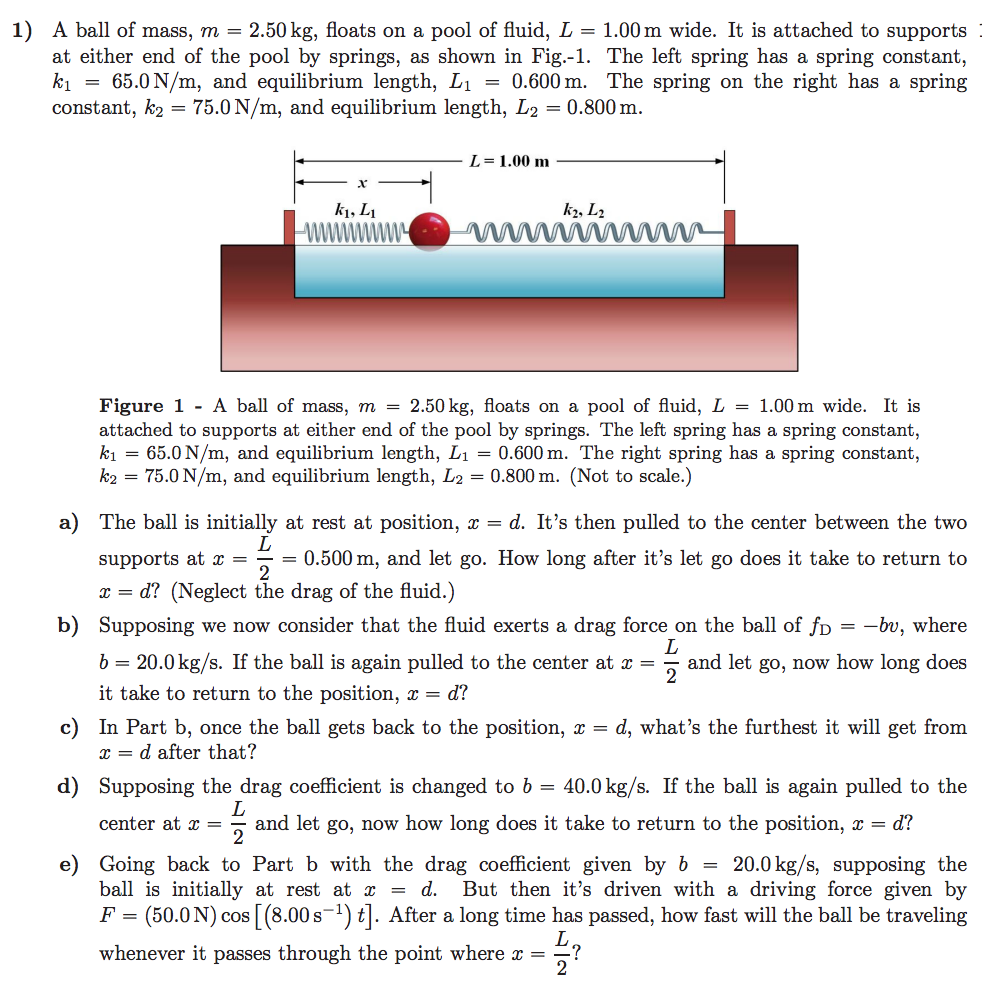# 1) A ball of mass, m = 2.50 kg, floats on a pool of fluid, L-1.00 m wide. It is attached to supportsat either end of the pool by springs, as shown in Fig.-1. The left spring has a spring constant,A = 65.0 N/m, and equilibrium length, L1-0.600 m. The spring on the right has a springconstant, k2-75.0N/m, and equilibrium length, L20.800mL = 1.00 mki, LiFigure 1 -A ball of mass, m = 2.50 kg, floats on a pool of fluid, L = 1.00 m wide. It isattached to supports at either end of the pool by springs. The left spring has a spring constant,A = 65.0 N/m, and equilibrium length, L1 = 0.600 m. The right spring has a spring constant.ka = 75.0 N/m, and equilibrium length, L2 = 0.800 m. (Not to scale.)a) The ball is initially at rest at position, x - d. It's then pulled to the center between the twosupports at 0.500 m, and let go. How long after it's let go does it take to return tox- d? (Neglect the drag of the fluid.)Supposing we now consider that the fuld exerts a drag force on the ball of fDb 20.0kg/s. If the ball is again pulled to the center at aand let go, now how long doesit take to return to the position, x = d?b)-bu, wherec) In Part b, once the ball gets back to the position, x - d, what's the furthest it will get fromr-d after that?d) Supposing the drag coefficient is changed to b = 40.0 kg/s. If the ball is again pulled to thecenter at and let go, now how long does it take to return to the position, x-d?2e) Going back to Part b with the drag coefficient given by b -20.0 kg/s, supposing theball is initially at rest at x - d. But then it's driven with a driving force given byF (50.0N) cos [(8.00s) ]. After a long time has passed, how fast will the ball be travelingwhenever it passes through the point where x?

Question
66 views

asking part D and E in another questionhelp_outlineImage Transcriptionclose1) A ball of mass, m = 2.50 kg, floats on a pool of fluid, L-1.00 m wide. It is attached to supports at either end of the pool by springs, as shown in Fig.-1. The left spring has a spring constant, A = 65.0 N/m, and equilibrium length, L1-0.600 m. The spring on the right has a spring constant, k2-75.0N/m, and equilibrium length, L20.800m L = 1.00 m ki, Li Figure 1 -A ball of mass, m = 2.50 kg, floats on a pool of fluid, L = 1.00 m wide. It is attached to supports at either end of the pool by springs. The left spring has a spring constant, A = 65.0 N/m, and equilibrium length, L1 = 0.600 m. The right spring has a spring constant. ka = 75.0 N/m, and equilibrium length, L2 = 0.800 m. (Not to scale.) a) The ball is initially at rest at position, x - d. It's then pulled to the center between the two supports at 0.500 m, and let go. How long after it's let go does it take to return to x- d? (Neglect the drag of the fluid.) Supposing we now consider that the fuld exerts a drag force on the ball of fD b 20.0kg/s. If the ball is again pulled to the center at aand let go, now how long does it take to return to the position, x = d? b) -bu, where c) In Part b, once the ball gets back to the position, x - d, what's the furthest it will get from r-d after that? d) Supposing the drag coefficient is changed to b = 40.0 kg/s. If the ball is again pulled to the center at and let go, now how long does it take to return to the position, x-d? 2 e) Going back to Part b with the drag coefficient given by b -20.0 kg/s, supposing the ball is initially at rest at x - d. But then it's driven with a driving force given by F (50.0N) cos [(8.00s) ]. After a long time has passed, how fast will the ball be traveling whenever it passes through the point where x? fullscreen
check_circle

Step 1

As per question, a block of mass m=2.5kg, is connected with the two spring, length of the pool L=1.0 m wide, the left spring has a spring constant k1=65.0 N/m has equilibrium length L1=0.600m, spring constant of right-hand spring k2=75.0 N/m length L2=0.800 m.

A. Ball is initially in the rest at x=d, and then pulled towards the center at x=L/2 position, then we have to find the time taken by the block to return to x=d?

B. When drag force is also working on the block then we have to find the time in which block returning to the x=d when it pulled to the x=L/2. Given that drag force fd= -bv where b=-20 kg/s.

C. In the case b, once ball gets back to position x=d, then we have to find the furthest distance covered by the block.

Step 2

As per the question, drag force is negligible and both the springs are connected in the parallel way,

So, spring equivalent

Step 3

Now, we know that time period of t...

### Want to see the full answer?

See Solution

#### Want to see this answer and more?

Solutions are written by subject experts who are available 24/7. Questions are typically answered within 1 hour.*

See Solution
*Response times may vary by subject and question.
Tagged in

### Vibrations